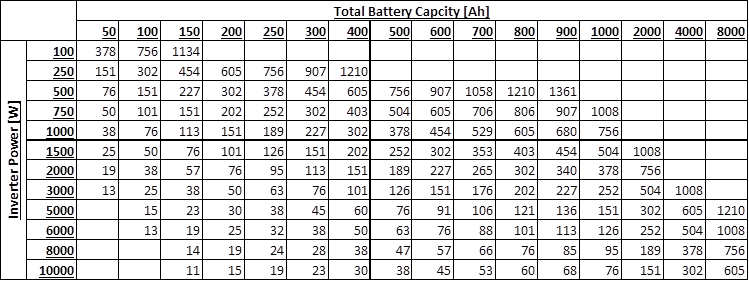One of the questions which come up quite often on some of my earlier articles about solar installations is, “how many batteries will I need?”. There are a couple of factors which you’ll need to consider when working out how many batteries you will need for your solar installation, but I’ve tried to simplify it as far as possible.

To start off, you’ll need to know what size inverter you’re going to be using, you’ll need it’s rated power in watts [W]. If you’re not yet sure what inverter you’re going to be using, here is a guide on selecting the correct inverter size for your solar or backup power system.

Next you’ll need to decide which table to work with, and this depends on how significant the load on your inverter is going to be during normal operation. If you expect your inverter to be running at near it’s rated power, so the continuous load on your inverter will be close to it’s rated power output, then use Table 1. The second table, Table 2, is more realistic for household use and is calculated with a continuous load of 60% of the inverter’s rated output. If you’re unsure then rather use Table 1, it will give you a more conservative estimate.

Lastly, you’ll need to know what the capacity of your batteries are and how long you’d like them to last for. A standard 12V deep cycle battery is usually 105Ah, this figure can be found on your battery data sheet or on the label on the battery. The duration in minutes is how long you’d like your batteries to last for. So if you’re going to be using the system in the evenings, then you’d probably want power from 5pm to 10pm, for a total of 5 hours which is 5 x 60 = 300 minutes. If it’s just for a short hour or two backup then you’ll only need them to last for 60-120 minutes.

In order to use the table, you start by looking for your inverter’s power in the left column. Say ours is 1000W and we’re working in Table 1. We then move along this row until we find the number of minutes closest to the figure which we calculated in the previous step, so 300 minutes is closest to 287 minutes. We then then move up this column to the battery capacity row, in this case we get 600Ah. This means that we require 600Ah worth of battery capacity to provide 1000W of inverter power for 5 hours. If we’re using 105Ah batteries then we would need 600 / 105 = 5.7, so 6 batteries of 105Ah to provide the required power.

We can do a similar example using Table 2, for operating at only 60% of the inverter’s rated power. Let’s  use the same figures from above and say our inverter’s power is still 1000W. We then move across the row to find the closest figure to 300 minutes, which is 302. We then move up the column to find the battery capacity and find 400Ah. This means that we’ll only need 4 batteries of 105Ah if we’re only running at 60% of the inverter’s rated power, rather than the 6 calculated using Table 1 at 100% of the inverter’s rated power.

### Table 1 – Operating At The Inverter’s Rated Power### Table 2 – Operating At 60% Of The Inverter’s Rated PowerSome additional notes on the figures calculated in the tables.

The inverter efficiency is assumed as 95% for Table 1, being a pure sine wave inverter operating at near it’s rated power output. The inverter efficiency in Table 2 is reduced to 90% as inverters are typically less efficient when producing power below their rated output.

In both cases the batteries are assumed to be discharged to 30% of their original capacity. This affects the life of the batteries, the deeper the discharge, the shorter the life of the battery. Some people prefer to design their systems to only allow for discharge to 50% or even 60% of their original capacity in order to extend the life of the batteries.

Do you have any tips, comments or suggestions? Please share them with us in the comments section below, we would love to hear from you.